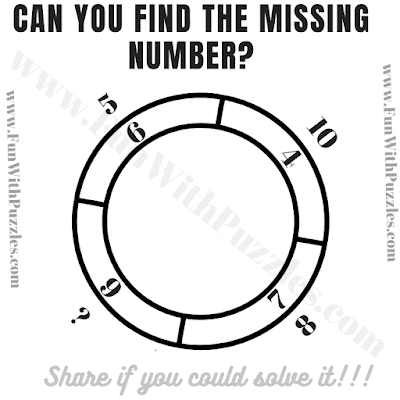This is a Math Logic Puzzle which will twist your brain. In this math brain teaser, you are shown some numbers inside and outside the double Circle. These numbers around these Circles relate to each other mathematically or logically. Your challenge in this math puzzle brain teaser is to crack this hidden code which relates these numbers in these Circles. If you are able to crack the code, find the value of the missing number which will replace the question mark.
This is a tough math logic puzzle. If you are able to solve it without looking at the answer, write down your answer in the comments along with your logical reasoning.Can you find the missing number?

The answer to this "Math Puzzle Brain Teaser", is hidden as of now. Please write down in comments in case you want to see the answer to this puzzle.

Unknown said...

Quite a fun puzzle.

Rajesh Kumar said...

Solving this puzzle is fun. However, this is a tough puzzle. Please write down your answer to this math puzzle along with your logical reasoning!

Lee Mowbray said...

The answer is 3. The difference between the top and bottom numbers are the same and the difference between the left and right numbers are the same.
Inner circle:
Top and bottom: difference between 6 and 4 is 2 and 7 and 9 is 2.
left and right: Difference between 6 and 9 is 3 and 4 and 7 is 3
Outer circle:
Top and bottom: difference between 5 and 10 is 5 and 8 and ? is 5
Left and right: difference between 10 and 8 is 2 and 5 and ? is 2
So the ? must equal 3: 8 - 3 = 5 and 5 - 3 = 2

Unknown said...

The adition of the direct opposite number in the inner circle is same as the addition of the direct opposite number in the outer circle.as for example (7+6)=(5+8)=13.in
the same way (9+4) =(10+3).so 3 should be the answer

Anonymous said...

4

Giddy said...

3. The sum of the outside circle is the same as the sum of the inner circle.